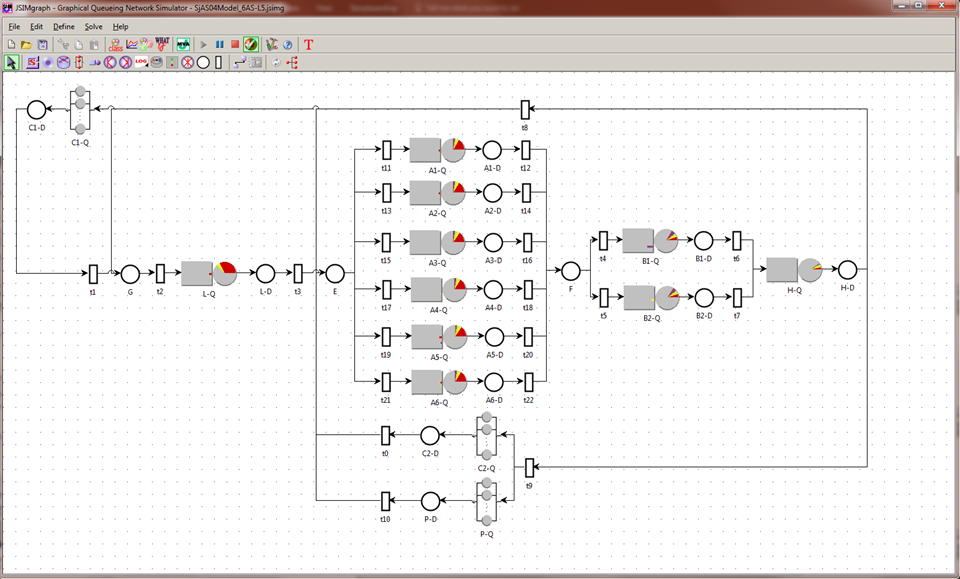# Software

### Java Modelling Tools

JMT is a performance modelling suite for capacity planning and system performance prediction. The suite is based on queueing network models. JMT features, among others, an advanced discrete-event simulator for queueing models and Petri nets  (jSIM) and mean value analysis algorithms (jMVA).### LINE

LINE is a MATLAB toolbox for solving extended and layered queueing network models. LINE can numerically compute percentiles of response times for service-level agreement (SLA) assessment, describe uncertainty about an operational environment using random environments, and solve queueing networks through model-to-model transformations to native algorithms (CTMC, Fluid ODEs, matrix-analytic methods, …) and external solvers (e.g., JMT, LQNS).

### KPC-Toolbox

KPC-Toolbox is a MATLAB toolbox for fitting empirical datasets into a class of stochastic processes known as Markovian Arrival Processes (MAPs). MAPs can fit non-exponential and long-range dependent time series data.  Given a time series, KPC-Toolbox automatically returns a MAP that fits statistical descriptors such as moments, correlations, and bi-correlations.